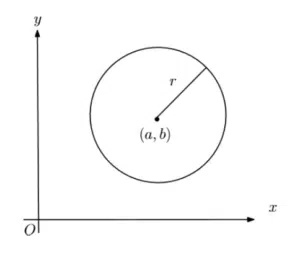# Equation of a Circle

## Equation of a Circle NotesWe find the equation of a circle from the coordinates of its centre and its radius. Consider this circle whose centre is at the point (a,b) and whose radius is r. The equation of the circle is given by

$(x-a)^2+(y-b)^2=r^2$.

The values of $a$, $b$ and $r$ are specified whereas $x$ and $y$ are left as general points. This is much like when we specify $m$ and $c$ in $y=mx+c$ when we have the equation of a given straight line. Note that the equation of a straight line is explicit – y is the subject and is given in terms of x. The circle equation, on the other hand, is implicit – the $x$s and $y$s are mixed up.

Take the Coordinate Geometry Course.

### Things to Learn for Circle Equation Questions

You should know the following facts:

1. Most importantly, the equation of a circle.
2. Secondly, that the angle subtended from a diameter at the circumference is a right angle.
3. Next, the radius and tangent touch at right angles to one another.
4.  Finally, a perpendicular from a chord bisects the chord.

You should be able to find the equation of a circle:

1. using completing the square and/or Pythagoras,
2. given a triangle that has all three points on its circumference and
3. find the equation of a tangent using perpendicular gradients.

Take the Coordinate Geometry Course.Dr Nat

### AS Mechanics SUVAT Equations

asmaths
5 Lectures
0 HoursDr Nat

### AS Maths Vectors

asmaths
15 Lectures
0 HoursDr Nat

### AS Maths Coordinate Geometry

asmaths
15 Lectures
0 HoursDr Nat

### AS Maths Binomial Expansion

asmaths
11 Lectures
0 HoursDr Nat

asmaths
17 Lectures
0 Hours